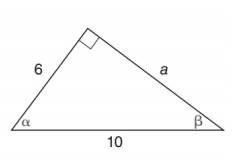Chapter 11.2, Problem 3EElementary Geometry For College St...

7th Edition
Alexander + 2 others
ISBN: 9781337614085

Solutions

Chapter
SectionElementary Geometry For College St...

7th Edition
Alexander + 2 others
ISBN: 9781337614085
Textbook Problem

In Exercises 1 to 6, find cos α and cos β .To determine

To find:

cosα and cosβ.

Explanation

Consider the following figure,

General formula for cosine ratio is given below,

From the given figure the value 10 is the hypotenuse value since it is opposite to right angle.

Then 6 is the length of the leg adjacent to α and a is the length of leg adjacent to β.

The Pythagorean theorem is given below,

(hypotenuse)2=(lengthofoneleg)2+(lengthofotherleg)2

Substitute the values of hypotenuse=10,lengthofoneleg=6 and lengthofotherleg=a to get the following,

Still sussing out bartleby?

Check out a sample textbook solution.

See a sample solution

The Solution to Your Study Problems

Bartleby provides explanations to thousands of textbook problems written by our experts, many with advanced degrees!

Get Started

In Problems 11-22, solve each system by elimination or by any convenient method.

Mathematical Applications for the Management, Life, and Social Sciences

x234x5

Applied Calculus for the Managerial, Life, and Social Sciences: A Brief Approach

For a = 2i + 3j − 4k and b = −i + 2j − k, a · b = 0 8 10 12

Study Guide for Stewart's Multivariable Calculus, 8th

If velocity is v(t) = 4t + 4 and s(1) = 2, then s(t) = 2t2 + 4t – 4 2t2 + 4t + 6 t2 + 4 t2 − 4

Study Guide for Stewart's Single Variable Calculus: Early Transcendentals, 8th